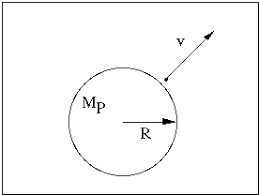# How to Calculate and Solve for Escape Velocity | The Calculator EncyclopediaThe image above represents the escape velocity.

To compute the escape velocity of a field, two essential parameters are needed and the parameters are acceleration due to gravity (g) and radius (r).

The formula for calculating the escape velocity:

V = √(2gR)

Where;
V = Escape velocity
g = Acceleration due to gravity

Let’s solve an example;
Find the escape velocity of a field when the acceleration due to gravity is 12 and the radius is 24 cm.

This implies that;
g = Acceleration due to gravity = 12
r = Radius = 24 cm

V = √(2gR)
V = √(2 x 12 x 24)
V = √(576)
V = 24

Therefore, the escape velocity is 24 m/s.

Nickzom Calculator – The Calculator Encyclopedia is capable of calculating the escape velocity.

To get the answer and workings of the escape velocity using the Nickzom Calculator – The Calculator Encyclopedia. First, you need to obtain the app.

You can get this app via any of these means:

To get access to the professional version via web, you need to register and subscribe for NGN 1,500 per annum to have utter access to all functionalities.
You can also try the demo version via https://www.nickzom.org/calculator

Once, you have obtained the calculator encyclopedia app, proceed to the Calculator Map, then click on Gravitational Field under the Physics sectionNow, Click on Escape Velocity under Gravitational FieldThe screenshot below displays the page or activity to enter your values, to get the answer for the escape velocity according to the respective parameters which are the acceleration due to gravity (g) and radius (r).Now, enter the values appropriately and accordingly for the parameters as required by the example above where the acceleration due to gravity (g) is 12 and radius (r) is 24 cm.Finally, Click on CalculateAs you can see from the screenshot above, Nickzom Calculator – The Calculator Encyclopedia solves for the escape velocity and presents the formula, workings and steps too.